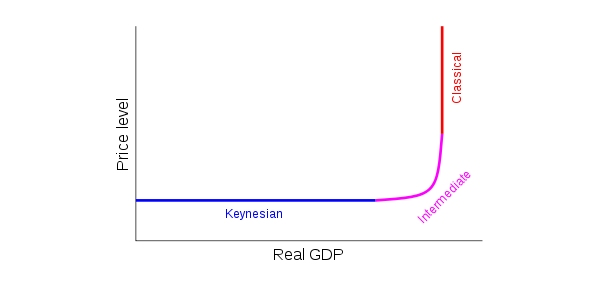# Aggregate Supply Trivia Quiz

19 Questions | Total Attempts: 103SettingsAggregate supply is the total supply of goods and services produced within an economy at a given overall price level in a given period. There is mostly a positive relationship between aggregate supply and price of an item. Test your understanding of this by taking up the questions below. All the best!

• 1.
Aggregate Supply is
• A.

The total amount of goods and services that all industries in the economy will produce at a given price level

• B.

A specific amount of goods and services that all industries in the economy will produce at a given price level

• C.

The total amount of goods and services that only one industry in the economy will produce at a given price level

• D.

A given amount of outputs that all industries in the economy will produce at a given price level.

• E.

The total amount of goods and services that only one industry in the economy will produce at any random price level.

• 2.
What is the relationship between the price level and the amount of output that a country's industries will supply?
• A.

Negative

• B.

Positive

• C.

No relationship

• 3.
The short run aggregate supply curve looks very much like a microeconomic supply curve that is ?
• A.

Downward sloping

• B.

Without sloping

• C.

Upward sloping

• 4.
In macroeconomics analysis, the short run is defined as
• A.

The period of time when the price of product do not change.

• B.

The period of time when the output levels of the production do not change

• C.

The period of time when the factors of production do not change.

• D.

The period of time when the prices of the factors of production do not change.

• E.

The period of time when the prices of the production changes.

• 5.
In a Aggregate Supply curve, the x-axis of the graph represents
• A.

Real output

• B.

Total output

• C.

National income

• D.

Price level

• E.

Demand quantity

• 6.
If a large level of output is produced, what average costs of production are the firms likely to face?
• A.

Normal average costs

• B.

Lower average costs

• C.

Higher average costs

• 7.
What is the most straightforward explanation of supply-side shocks?
• A.

They are changes in the cost of production.

• B.

They are factors that cause decreasing cost of production.

• C.

They are factors that cause increasing cost of production.

• D.

It is not related to cost of production.

• E.

Both B &C.

• 8.
A ___ in wages will result in an ____ in the cost of production to firms, and therefore a fall in aggregate demand.
• A.

Increase; decrease

• B.

Increase; increase

• C.

Decrease; decrease

• D.

Decrease; increase

• E.

None of above

• 9.
Changes in the costs of production include:
• A.

A change in wage rates

• B.

A change in the costs of raw materials

• C.

A change in the price of imports

• D.

All above

• 10.
The economy will operate where aggregate demand is equal to aggregate demand.
• A.

False

• B.

True

• 11.
Two main Schools of thought concerning the shape of the Long Run Aggregate Supply are
• 12.
In Keynesian LRAS supply curve phase 3 (where the slope is biggest), when the economy reaches its full capacity it is impossible to increase output any further because factors of production are fully employed, this suggest that the LRAS is _____.
• A.

Perfectly elastic

• B.

Elastic

• C.

Perfectly inelastic

• D.

Inelastic

• 13.
Does full employment mean that there is no unemployment at all?
• A.

Yes.

• B.

No, it only indicates that the unemployment rate is below 5%.

• C.

No, it indicates the unemployment rate is somewhere around 10%

• 14.
In Neo Classical LRAS curve, when the price level rise, does the level of output change?
• A.

No.

• B.

Yes.

• 15.
In Keynesian perspective:
• A.

Decrease aggregate demand without the need of supply side policies, when starting from a position of low demand.

• B.

Increase aggregate demand with the need of supply side policies, when starting from a position of high demand.

• C.

Decrease aggregate demand without the need of supply side policies, when starting from a position of low demand.

• D.

Increase aggregate demand without the need of supply side policies, when starting from a position of low demand.

• E.

None of above

• 16.
What will cause the LRAS curve shift to the right?
• A.

• B.

Improvements in educations

• C.

Discoveries of new resources

• D.

All above

• 17.
Supply side policies can be divided into two categories:
• A.

Market oriented policies

• B.

Interventionist policies

• C.

Monetary policies

• D.

Fiscal policies

• E.

Both a and b

• 18.
Market Oriented supply side policies does not include
• A.

Reduction in income taxes

• B.

Deregulation

• C.

Privatization

• D.

• E.

Increase in corporation taxes

• 19.
Interventionist supply side policies include:
• A.

Education and training

• B.

Research and development

• C.

Provision of infrastructure

• D.

Improved information

• E.

All above

Related TopicsBack to top